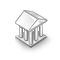거래 로봇을 무료로 다운로드 하는 법을 시청해보세요
스크립트가 흥미로우신가요?
그렇다면 링크 to it -
하셔서 다른 이들이 평가할 수 있도록 해보세요
스크립트가 마음에 드시나요? MetaTrader 5 터미널에서 시도해보십시오# Function ObjectGetValue_ByCurrent .....Delta_ByCurrent .....Delta_ByTimeShift .....Delta_PerBar - MetaTrader 4용 라이브러리

조회수:
5508
평가:
투표: 7
게시됨:
2008.05.16 06:39
업데이트됨:
2016.11.22 07:32

The functions offered here return the prices that linearly change with the time for a graphical object located in any chart window.

The functions are located in the body of a test script that visually demonstrates how a bar is being formed.

Concept.

Standard function ObjectGetValueByShift() returns the values in the points of bar opening time, i.e., discretely with the timeframe step.

This is determined by passing of the parameter of the chart X-coordinate as (int) of the bar number.

Calling to the standard function ObjectGetValueByShift() within the bar forming time frame, we obtain the same value.

Standard function ObjectGetValueByShift() does not calculate the changes of Y-coordinate within the entire period of bar forming.

When creating ATSes that response to any intersections of the graphical object, namely of TrendLine, the "error" of tens of points may be accumulated.

Correspondingly, we will need some functions that would return the true value at every tick.

```ObjectGetDelta_PerBar(string "ObjectName")

ObjectGetDelta_ByCurrent (string "ObjectName")

ObjectGetValue_ByCurrent(string "ObjectName", int BarNumber)

ObjectGetDelta_ByTimeShift(string "ObjectName", double TimeShift   )```

Function ObjectGetDelta_PerBar(string "ObjectName") returns the slant of a graphical object by 1 bar as calculated from the object coordinates, i.e., it returns delta = one-bar increment. Calculation features. Standard function iBarShift() returns zero for all times set to the right of zero bar. However, a graphical object may be located to the right of zero bar. This is why the length of the graphical object in bars is calculated as the difference between the object coordinates times, the result being divided by the timeframe. This allows us to operate with objects that step out of zero bar position.

Function ObjectGetDelta_ByCurrent (string "ObjectName") claculates the current value inside a bar by the time elapsed since the bar began.

Function ObjectGetValue_ByCurrent(string "ObjectName", int BarNumber ) sums up the value of the current linear increment ObjectGetDelta_ByCurrent () and the value at the preset bar number, if this value isn't equal to zero.

This will be a true value for zero bar. However, on any other bar, this will be the values shifted by the time taken by zero-bar forming. I.e., if you call it with a bar number other than zero, it will show a smooth shift proportional to the time taken by zero-bar forming. In our opinion, this feature is convenient for the sake of ATS.

A zero value of the graphical object's price is obtained outside its range. In this case, the function will return zero. The programmer must check the return value for non-being equal to zero.

ObjectGetDelta_ByTimeShift(string "ObjectName", double TimeShift )

To build an ATS, it is convenient to have a function that would give you the value of Y-coordinate increment by a preset time, шт the past or in the future.

This script, when tested on demo/real М1 charts, demonstrates:

1. irregularity of incoming ticks;

2. delay of bar opening/drawing event due to no ticks;

3. temporarily absent bars and post-factum assigning their opening times to them.

For example:

Time=1208396460 line=162.5545 current value within bar 0=162.5300 line (- 1)=162.5273 delta by (1) bar=-0.0273 delta by (2) bars=-0.0545

Bar time percentage=72% correction for price line=-0.0195

Time=1208396400 line=162.5818 current value within bar 0=162.5400 line (- 1)=162.5545 delta by (1) bar=-0.0273 delta by (2) bars=-0.0545

Bar time percentage=152% correction for price line=-0.0414

Time=1208396400 line=162.5818 current value within bar 0=162.5500 line (- 1)=162.5545 delta by (1) bar=-0.0273 delta by (2) bars=-0.0545

Bar time percentage=127% correction for price line=-0.0345

Recommendations:

Explanation: See results in "Expert Advisors" at the bottom, near "Journal".

When removing the script from a chart, there can be a delay produced by MT 4 taking some time for termination.

MetaQuotes Software Corp.에서 러시아어로 번역함.
원본 코드: https://www.mql5.com/ru/code/8080GTerminal_V5 Graphic orders and indicators

Execution of orders by slanting lines. For the purposes of real trading and learning, graphical orders are implemented in Tester. The EA was evaluated on MQL4 Forum. Version_V5 considers all the suggestions and wishes.Halifax

Leading Edge trend estimator with bands.FX-CHAOS

Draft EA that works on H4, first entering on daily chart. It uses Ryan Jones' Fixed Ratio money management method and "reverse pyramid.Pattern Searching ZigZag

Building in the current window a ZigZag calculated on a larger timeframe.